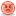##Use timer to determine enemy attack patterns

Hi guys,

I've developing a 2D shoot em up game where I'm using a timer to increase the "Level" as the game progresses to determine how enemies are spawned on the screen - Level 1 = spawns 1 enemy, level 2 = spawns 2 enemies, etc. I just wondering if anyone knew of a way to code it so that as the level increases requirements/properties would increase too such as the timer by 30 seconds, enemies are faster, etc.
Actionscript Code:
`public class MainShapeShooter14 extends MovieClip   {public var level:int = 1;public var enemies:Array;var _timer:Timer;public function MainShapeShooter14()    { level = 1;_timer = new Timer(5000);enemies = new Array(); makeEnemies();}public function makeEnemies():void{   _timer.start();   for(var i:int = 0; i < level; i++){     var tempEnemy:Enemy = new Enemy();     addChild(tempEnemy);    var randomXPosition = Math.round(Math.random() * 100 - 200);    var randomXYPosition = Math.round(Math.random() * -100 - -200);    var randomYPosition = Math.round(Math.random() * 400 || -100);    tempEnemy.x = randomXPosition;    tempEnemy.xy = randomXYPosition;     tempEnemy.y = randomYPosition;    enemies.push(tempEnemy);    tempEnemy.gotoAndStop(1);tempEnemy.addEventListener(Event.ENTER_FRAME, onEnemyMove); _timer.addEventListener(TimerEvent.TIMER, onUpdateTime);  }}function onEnemyMove(event:Event):void{    var enemy:Enemy = Enemy(event.currentTarget);     var xDiff:Number = player.x - enemy.x;     var yDiff:Number = player.y - enemy.y;    //if they are further than maxEnemyStep away in x     if (Math.abs(xDiff) > maxEnemyStep){        if (xDiff < 0){          xDiff = -maxEnemyStep;        }else{          xDiff = maxEnemyStep;        }     }     //if they are further than maxEnemyStep away in y     if (Math.abs(yDiff) > maxEnemyStep){        if (yDiff < 0){          yDiff = -maxEnemyStep;        }else{          yDiff = maxEnemyStep;        }     }     enemy.x += xDiff;     enemy.y += yDiff;     xDiff *= _friction;     yDiff *= _friction;     enemy.gotoAndStop(1);   }    } function onUpdateTime(event:Event):void{                level ++;                trace ("Level" + level);                levelText.text = level.toString();                          _timer.reset();                makeEnemies();                    }`

Thank you for your time and reading all that and any help/suggestions would be greatly appreciated.

Jonesy